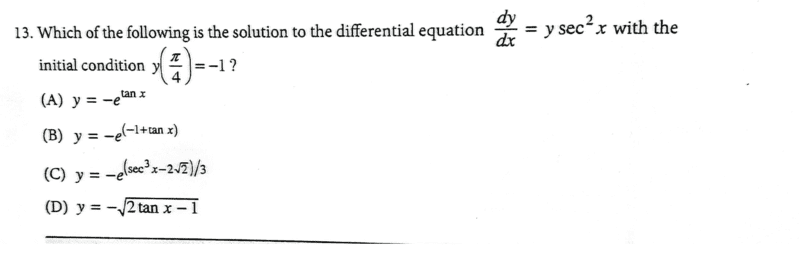# Solve the given first order differential equation

• chwala
In summary, the conversation discusses the use of separation of variables to solve a first order differential equation, but the initial condition provided leads to an incorrect solution. The correct solution is found by substituting the initial condition into the general solution obtained from separation of variables. However, it is noted that the question does not specify which method should be used to solve the equation and therefore the work would not be marked as wrong.

#### chwala

Gold Member
Homework Statement
This is a text question-
Relevant Equations
separation of variables
first order differential equation- integrating factor...My thinking is two-fold, firstly, i noted that we can use separation of variables; i.e

##\dfrac{dy}{y}= \sec^2 x dx##

on integrating both sides we have;

##\ln y = \tan x + k##

##y=e^{\tan x+k} ##

now i got stuck here as we cannot apply the initial condition ##y(\dfrac {π}{4})=-1##

Secondly on using;

##\dfrac{dy}{dx}+ P(x)y=q(x)##

i have

##\dfrac{dy}{dx}-\sec^2 x=0##

i.f= ##e^{-\int sec^2x dx} =e^{-\tan x}##

therefore,

##(e^{-\tanx }⋅y)' =0## on integration, we shall have;

##(e^{-\tan x} ⋅y) =k## now using the initial condition, ##y(\dfrac {π}{4})=-1##

we have, ##k=-\dfrac{1}{e}##

thus,
##y=e^{\tan x} ⋅k=\left[ e^{\tan x} ⋅-\dfrac{1}{e}\right]=-e^{\tan x-1}##

i do not have solution to the problem...your insight is welcome...

Last edited:
I don't see what your problem is. You found that $$y=-e^{\tan(x) -1}$$

•chwala
chwala said:
on integrating both sides we have;
##\ln y = \tan x + k##

##y=e^{\tan x+k} ##

now i got stuck here as we cannot apply the initial condition ##y(\dfrac {π}{4})=-1##
Your second equation above is equivalent to ##y = e^{\tan(x)}\cdot e^k## or ##y = Ce^{\tan(x)}##, where ##C= e^k##. Simply substitute ##\pi/4## for x and -1 for y to solve for C.

•WWGD and chwala
chwala said:
Homework Statement:: This is a text question-
Relevant Equations:: separation of variables
first order differential equation- integrating factor...

View attachment 319644

My thinking is two-fold, firstly i noted that we can use separation of variables; i.e

##\dfrac{dy}{y}= \sec^2 x dx##

on integrating both sides we have;

##\ln y = \tan x + k##

##y=e^{\tan x+k} ##

now i got stuck here as we cannot apply the initial condition ##y(\dfrac {π}{4})=-1##
Keep in mind:

##\displaystyle \int \dfrac{dy}{y} = \ln |y| + C ##

•malawi_glenn and chwala
phyzguy said:
I don't see what your problem is. You found that $$y=-e^{\tan(x) -1}$$

Correct...

My question is 'would a student be wrong in attempting to use separation of variables?' ...does he/she get method marks? cheers...

Mark44 said:
Your second equation above is equivalent to ##y = e^{\tan(x)}\cdot e^k## or ##y = Ce^{\tan(x)}##, where ##C= e^k##. Simply substitute ##\pi/4## for x and -1 for y to solve for C.
Won't we have ##e^k=-1##?

chwala said:
Won't we have ##e^k=-1##?
No. Solve for C in this equation: ##-1 = Ce^1##

•chwala
chwala said:
My question is 'would a student be wrong in attempting to use separation of variables?' ...does he/she get method marks?
I don't see why the work would be marked as wrong. The question asks only which of the four listed equation is the solution and makes no requirement of which method to use.

Thanks @Mark44 ...my interest was on checking if we could use separation of variables. You have clearly shown that to me. Cheers!

Last edited:
•WWGD